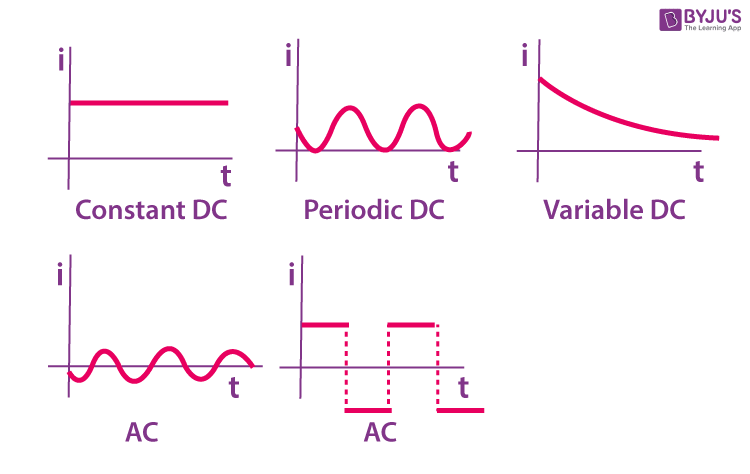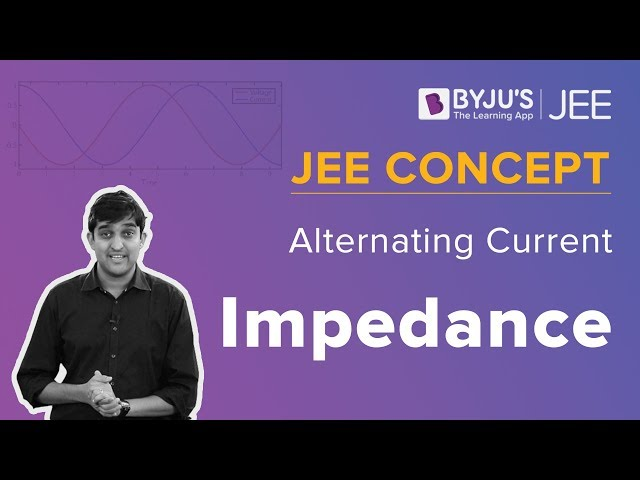Win up to 100% scholarship on Aakash BYJU'S JEE/NEET courses with ABNAT Win up to 100% scholarship on Aakash BYJU'S JEE/NEET courses with ABNAT

# Alternating Current IIT JEE Study Material

An Alternating Current (AC) changes its direction periodically, whereas if the direction of the current is constant, then it is called a direct current (DC). Let us consider a sinusoidal varying function:

$$\begin{array}{l}\mathbf{i\;=\;I_{m}\;sin\;(\omega t\;+\;\Phi )}\end{array}$$
Here, the maximum current (peak current) is denoted by Ιm and ‘i’ is the instantaneous current. The factor (ωt + Φ) is called phase, and ω is termed angular frequency. In terms of frequency,
$$\begin{array}{l}\mathbf{\omega \;=\;2\;\pi \;f}\end{array}$$
Also,
$$\begin{array}{l}\text{frequency}\ \mathbf{f\;=\;\frac{1}{Time\;Period\;(T)}}\end{array}$$## Average Value

It is the average of all the instantaneous values of an AC (alternating current) and alternating voltages over 1 full cycle. The +ve half cycle will be exactly equal to the -ve half cycle in symmetrical waves like voltage or sinusoidal current waveform. Therefore, the average value of a complete cycle will always be 0. The work is done by both the + ve and -ve cycles. Therefore, the average value is calculated without taking its sign into consideration. Therefore, the only +ve half cycle is taken into consideration in determining the average value of alternating quantities (sinusoidal waves).

The average value for one alternation 0 → π is

$$\begin{array}{l}\mathbf{i_{avg}\;=\;\frac{i_{1}\;+\;i_{2}\;+\;i_{3}\;+\;.\;.\;.\;.\;+\;i_{n}}{n}}\end{array}$$

The mean or average value of a given function over a specified time interval between t1 and t2 is determined by:

$$\begin{array}{l}\mathbf{F_{av}\;=\;\frac{1}{t_{2}\;-\;t_{1}}\;\;\int_{t_{1}}^{t_{2}}\;\;f(t)\;dt}\end{array}$$

The cycle of a periodic function is continuously repeated, irrespective of its shape. For example, sinusoidal function. The average value of such periodic function is determined by:

$$\begin{array}{l}\mathbf{F_{av}\;=\;\frac{1}{T}\;\int_{0}^{T}\;\;f(t)\;dt}\end{array}$$

Where, T = time period of the periodic function.

For a symmetrical AC or alternating current (either sinusoidal or nonsinusoidal), the average value is calculated by taking only the mean or average of one alternation or one-half cycle. Therefore, for all the symmetrical waveforms,

$$\begin{array}{l}\mathbf{F_{av}\;=\;\frac{1}{\frac{T}{2}}\;\int_{0}^{T}\;\;f(t)\;dt}\end{array}$$

## Root Mean Square (RMS) Value

Split the base of 1 alternation into ‘n’ equal parts. Let i1, i2,…., in be the ordinates of ‘n’ equal parts, then,

Heat generated during first interval

$$\begin{array}{l}=\mathbf{i_{1}^{2}\;R\; \times \;\frac{T}{n}\;\;J}\end{array}$$

Heat generated during second interval

$$\begin{array}{l}=\mathbf{i_{2}^{2}\;R\; \times \;\frac{T}{n}\;\;J}\end{array}$$

Heat generated during third interval

$$\begin{array}{l}=\mathbf{i_{3}^{2}\;R\; \times \;\frac{T}{n}\;\;J}\end{array}$$

Heat generated during nth interval

$$\begin{array}{l}=\mathbf{i_{n}^{2}\;R\; \times \;\frac{T}{n}\;\;J}\end{array}$$

Total heat generated in time T

$$\begin{array}{l}=\mathbf{R\;T\;\left [ \frac{i_{1}^{2}\;+\;i_{2}^{2}\;+\;i_{3}^{2}\;+\;.\;.\;.\;+\;i_{n}^{2}}{n} \right ]\;\;J}\end{array}$$

If Ieff is the effective current, then the heat generated by this current in time

$$\begin{array}{l}\mathbf{T\;=\;I_{eff}^{2}\;\;R\;T }\end{array}$$
Joules. Also, by definition, both these expressions are equal.

i.e.,

$$\begin{array}{l}\mathbf{I_{eff}^{2}\;\;R\;T\;=\;R\;T\;\left [ \frac{i_{1}^{2}\;+\;i_{2}^{2}\;+\;i_{3}^{2}\;+\;.\;.\;.\;+\;i_{n}^{2}}{n} \right ] }\end{array}$$

Or,

$$\begin{array}{l}\mathbf{I_{eff}^{2}\;=\;\left [ \frac{i_{1}^{2}\;+\;i_{2}^{2}\;+\;i_{3}^{2}\;+\;.\;.\;.\;+\;i_{n}^{2}}{n} \right ] }\end{array}$$

Or,

$$\begin{array}{l}\mathbf{I_{eff}\;=\;\sqrt{ \frac{i_{1}^{2}\;+\;i_{2}^{2}\;+\;i_{3}^{2}\;+\;.\;.\;.\;+\;i_{n}^{2}}{n}}}\end{array}$$

The virtual or effective value of an alternating voltage or current is equal to the square root of the mean or average of the squares of the successive ordinates. Therefore, it is termed the root mean square or RMS value.

The effective or RMS (root mean square) value over a time period (T) of an alternating quantity is determined by:

$$\begin{array}{l}\mathbf{F_{rms}\;=\;\sqrt{\frac{1}{T}\;\;\int_{0}^{T}\;F^{2}\;(t)\;dt}}\end{array}$$

## Power Consumed

In an LCR circuit connected in series,

Voltage, V = V0sinωt

Let the electric current flowing through it be,

I = I0sin (ωt +Φ)

Instantaneous power, P = VI

=  (V0sinωt) (I0sin (ωt +Φ))

= (1/2)2(V0sinωt) (I0sin (ωt +Φ))

= (1/2) V0I0 (cos Φ – cos (2ωt + Φ))

The average value of cos (2ωt + Φ) over a complete cycle is 0

The average power consumed in a cycle:

Pav = (1/2) V0I0 cosΦ

$$\begin{array}{l}=\frac{V_{0}}{\sqrt{2}}\frac{I_{0}}{\sqrt{2}}cos\phi\end{array}$$

Here,

$$\begin{array}{l}\mathbf{cos\;\Phi \;=\;Power\;Factor}\end{array}$$

## RC Circuit in Series with an AC Source

$$\begin{array}{l}\mathbf{I_{m}\;=\;\frac{V_{m}}{\sqrt{X_{c}^{2}\;+\;R^{2}}}}\end{array}$$

And,

$$\begin{array}{l}\mathbf{tan\;\Phi \;=\;\frac{I_{m}\;X_{C}}{I_{m}\;R}\;=\;\frac{X_{C}}{R}}\end{array}$$

## LR Circuit in Series with an AC Source

$$\begin{array}{l}\mathbf{V\;=\;\sqrt{\left ( IR \right )^{2}\;+\;\left ( IX_{L} \right )^{2}}\;=\;I\;\sqrt{R^{2}\;+\;X_{L}^{2}}\;=\;IZ}\end{array}$$

Where,

$$\begin{array}{l}\text{Impedance}\ \mathbf{Z\;=\;\sqrt{R^{2}\;+\;X_{L}^{2}}}\end{array}$$

And,

$$\begin{array}{l}\mathbf{tan\;\Phi \;=\;\frac{I\;X_{L}}{I\;R}\;=\;\frac{X_{L}}{R}}\end{array}$$

## LC Circuit in Series with an AC Source

$$\begin{array}{l}\mathbf{V\;=\;I\;\left | X_{L}\;-\;X_{C} \right |\;=\;IZ\;\;and\;\;\Phi \;=\;90}\end{array}$$

## RLC Circuit in Series with an AC Source

$$\begin{array}{l}\mathbf{V\;=\;\sqrt{\left ( IR \right )^{2}+\left ( IX_{L} \;-\;IX_{C} \right )^{2}}\;=\;I\;\;\sqrt{R^{2}\;+\;\left ( X_{L}\;-\;X_{C} \right )^{2}}\;=\;I\;Z}\end{array}$$

Where,

$$\begin{array}{l}\mathbf{Z\;=\;\sqrt{R^{2}\;+\;\left ( X_{L}\;-\;X_{C} \right )^{2}}}\end{array}$$

And,

$$\begin{array}{l}\mathbf{tan\;\Phi \;=\;\frac{I\;\left ( X_{L} -X_{C} \right )}{IR}\;=\;\frac{X_{L}\;-\;X_{C}}{R}}\end{array}$$

## Resonance:

In a series RLC circuit, there becomes a point of frequency where the inductive reactance of the inductor becomes equal to the capacitive reactance of the capacitor. In other words, Resonance occurs when XL = XCThe point at which this occurs is called the resonance frequency point.

The amplitude of current in an RLC circuit is maximum for a given value of R and V if the impedance of the circuit is minimised. This is the condition for resonance.

Or,

$$\begin{array}{l}\mathbf{2\;\pi \;f\;L\;=\;\frac{1}{2\;\pi \;f\;C}}\end{array}$$

Or,

$$\begin{array}{l}\mathbf{f^{2}\;=\;\frac{1}{2\;\pi\;L\; \times \;2\;\pi \;C}\;=\;\frac{1}{4\;\pi ^{2}\;L\;C}}\end{array}$$

Or,

$$\begin{array}{l}\mathbf{f\;=\sqrt{\frac{1}{4\;\pi ^{2}\;L\;C}}\;=\;\frac{1}{2\;\pi \;\sqrt{LC}}\;\;Hz}\end{array}$$

Therefore,

$$\begin{array}{l}\mathbf{\omega _{r} \;=\;\frac{1}{\sqrt{LC}}}\end{array}$$

## Alternating Current – JEE Concept## Alternating Current Important JEE Questions## Frequently Asked Questions on Alternating Current

Q1

### Why is AC preferred over DC?

Alternating current (AC) is preferred over direct current (DC) because of the following reasons:
Alternating current is economical.
It is easier to produce alternating current.
It can be easily transferred from one place to another.
It can be easily stepped up and stepped down.

Q2

### Why is alternating current more dangerous?

The frequency of the alternating current is about 60 cycles per second and has a severe effect on the human body. At this frequency, even a small voltage of 25 volts can kill a person.

Q3

### Why is alternating current not used in electrolysis?

The alternating current is not used in electrolysis because the polarity of the electrodes keeps changing, which will affect electrolysis.

Q4

### Is alternating current always sinusoidal?

The alternating current produced by the rotating machinery will be a sinusoidal wave. However, non-sinusoidal alternating current can also be generated.